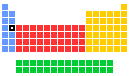▸▸
• 🇬🇧 Calcium
• 🇺🇦 Кальцій
• 🇨🇳 鈣
• 🇳🇱 Calcium
• 🇫🇷 Calcium
• 🇩🇪 Calcium
• 🇮🇱 סידן
• 🇮🇹 Calcio
• 🇯🇵 カルシウム
• 🇵🇹 Cálcio
• 🇪🇸 Calcio
• 🇸🇪 Kalcium
• 🇷🇺 Кальций

# Calcium: reactions of elements20Ca

## Reaction of calcium with air

Calcium is a silvery white metal. The surface of calcium metal is covered with a thin layer of oxide that helps protect the metal from attack by air, but to a lesser extent than the corresponding layer in magnesium. Once ignited, calcium metal burns in air to give a mixture of white calcium oxide, CaO, and calcium nitride, Ca3N2. Calcium oxide is more normally made by heating calcium carbonate. Calcium, immediately below magnesium in the periodic table is more reactive with air than magnesium.

2Ca(s) + O2(g) → 2CaO(s)

3Ca(s) + N2(g) → Ca3N2(s)

## Reaction of calcium with water

Calcium reacts slowly with water. This is in contrast with magnesium, immediately above calcium in the periodic table, which is virtually unreactive with cold water. The reaction forms calcium hydroxide, Ca(OH)2 and hydrogen gas (H2). The calcium metal sinks in water and after an hour or so bubbles of hydrogen are evident, stuck to the surface of the metal.

Ca(s) + 2H2O(g) → Ca(OH)2(aq) + H2(g)

## Reaction of calcium with the halogens

Calcium is very reactive towards the halogens fluorine, F2, chlorine, Cl2 bromine, Br2, or iodine, I2, and burns to form the dihalides calcium(II) fluoride, CaF2 , calcium(II) chloride, CaCl2, calcium(II) bromide, CaBr2, and calcium(II) iodide, CaI2 respectively. The reactions with bromine and iodine require heat to enable the formation of the products.

Ca(s) + F2(g) → CaF2(s)

Ca(s) + Cl2(g) → CaCl2(s)

Ca(s) + Br2(g) → CaBr2(s)

Ca(s) + I2(g) → CaI2(s)

## Reaction of calcium with acids

Calcium metal dissolves readily in dilute or concentrated hydrochloric acid to form solutions containing the aquated Ca(II) ion together with hydrogen gas, H2.

Ca(s) + 2HCl(aq) → Ca2+(aq) + 2Cl-(aq) + H2(g)